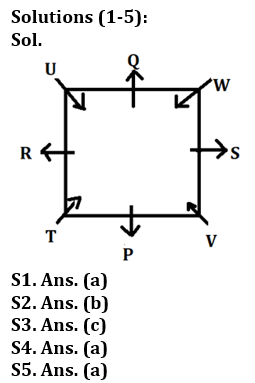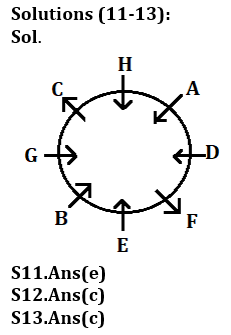Latest Banking jobs   »

# Reasoning Quiz For Bank of Baroda AO 2023-17th March

Directions (1-5): Study the following information carefully and answer the questions given below:
Eight persons P, Q, R, S, T, U, V and W are sitting on a square table such that some persons are sitting on the corners and some persons are sitting in middle of the table. The one who sits at the corner are facing towards the centre of the table and the one who sits at the middle are facing outside the centre of table but not necessarily in the same order. Q and V are not immediate neighbours of each other. T sits immediate right of P. P is facing outside the centre table and one person sits between P and R. Two persons sit between R and V. S sits immediate right of V. One person sits between V and W. W and P are not immediate neighbours of each other. U and Q are immediate neighbours of each other. Q is not facing the centre of the table.

Q1. Who among the following person sits immediate left of T?
(a) R
(b) U
(c) Q
(d) W
(e) None of these

Q2. Four of the following five are alike in a certain way and hence form a group find the one who does not belong to that group?
(a) T
(b) P
(c) V
(d) W
(e) U

Q3. If P and Q interchange their position then who sits immediate right of Q?
(a) W
(b) S
(c) T
(d) R
(e) None of these

Q4. Who among the following person sits immediate left of W?
(a) S
(b) V
(c) U
(d) P
(e) None of these

Q5. Who among the following person is facing U?
(a) V
(b) S
(c) W
(d) T
(e) None of these

Directions (6-10): In each of the question, relationships between some elements are shown in the statements. These statements are followed by conclusions numbered I and II. Read the statements and give the answer.
(a) If only conclusion I follows.
(b) If only conclusion II follows.
(c) If either conclusion I or II follows.
(d) If neither conclusion I nor II follows.
(e) If both conclusions I and II follow.

Q6. Statements: C>V≥B<N<M>A>S>D>F>G
Conclusion: I: V>S
II: V≤S

Q7. Statements: Z<X<C≤V; B>C<G<H≤J
Conclusion: I: Z<G
II: X<J

Q8. Statements: A>S>D>F>G<H<J<K<L
Conclusion I: A>G
II: H<L

Q9. Statements: X>C>H≥J≥K; H>Y≥U<I<O
Conclusion. I:X>U
II: K<I

Q10. Statements: Z>X>C<V; X>A>S<D<F; D>Q<W<R
Conclusion I: Z>W
II: V<R

Directions (11-13): Study the information carefully and answer the questions given below.
Eight persons are sitting in a circular table in such a way that some are facing towards center and some are facing outside the center. D sits second to the right of E, who faces towards the center. B sits third to the left of D. C sits opposite to F. One person sits between H and G. H and C sits immediate right to each other’s. H does not sit opposite to the one who sits immediate left of A. A faces towards center. B and G face same direction to H. F face same direction to C. C does not sit immediate left of E.

Q11. Who among the following sits second to the left of A?
(a) D
(b) G
(c) B
(d) H
(e) None of these

Q12. How many persons sit between C and E, when counted to the left of C?
(a) Three
(b) One
(c) Two
(d) Four
(e) None

Q13. How is B related to H?
(a) Second to the left
(b) Immediate right
(c) Third to the right
(d) Fourth to the left
(e) None of these

Direction (14-15): In these questions, relationship between different elements is show in the statements. The statements are followed by conclusions. Study the conclusions based on the given statements and select the appropriate answer:
(a) If only conclusion I follows.
(b) If only conclusion II follows.
(c) If either conclusion I or II follows
(d) If neither conclusion I nor II follows.
(e) If both conclusions I and II follow.

Q14. Statements: A≤M<T≤B=N>R>Y≥P>L
Conclusion I: M≤N II: L<B

Q15. Statements: K≥V=E≥M=X≤W>L≥S
Conclusion I: K≥X II: W>V

SolutionsSolutions (6-10):
S6. Ans.(c)
Sol. I: V>S (False) II: V≤S (False)

S7. Ans.(e)
Sol. I: Z<G (True) II:X<J (True)

S8. Ans.(e)
Sol. I: A>G (True) II:H<L (True)

S9. Ans.(a)
Sol. I:X>U (True) II: K<I (False)

S10. Ans.(d)
Sol. I: Z>W (False) II: V<R(False)Solutions (14-15):
S14. Ans(b)
Sol. I: M≤N(False) II: L<B(True)

S15. Ans(a)
Sol. I: K≥X (True) II: W>V (False)## FAQs

### What is the selection process of Bank of Baroda AO 2023?

The selection process of Bank of Baroda AO consists of Online exam and Interview

#### Congratulations!Union Budget 2023-24: Free PDF